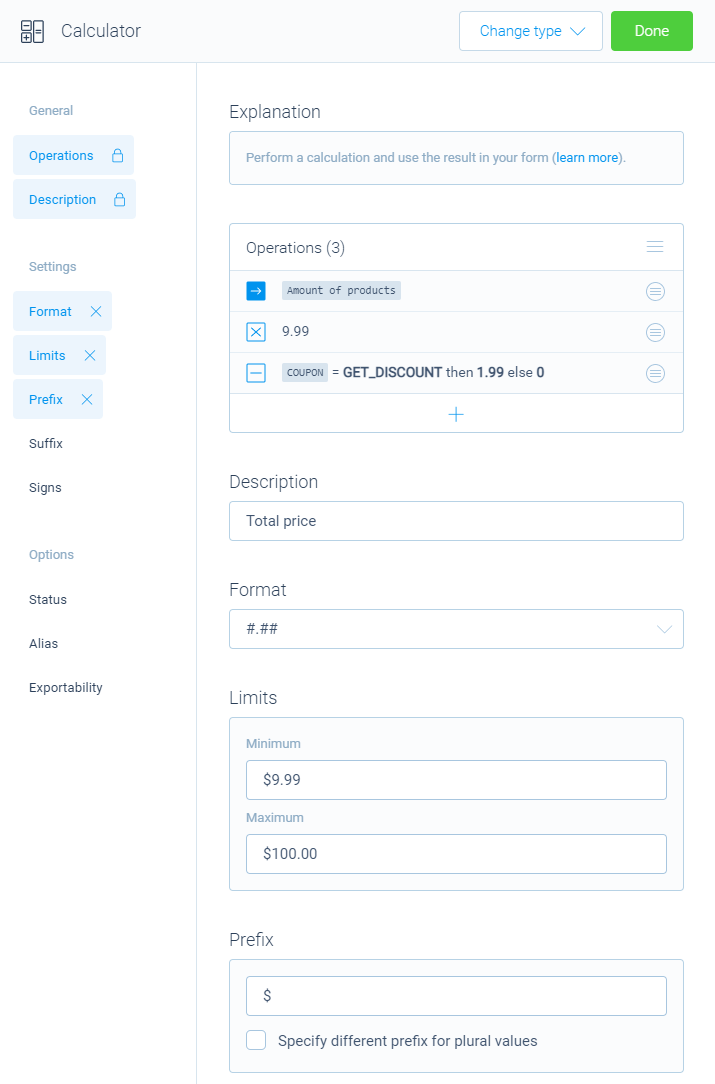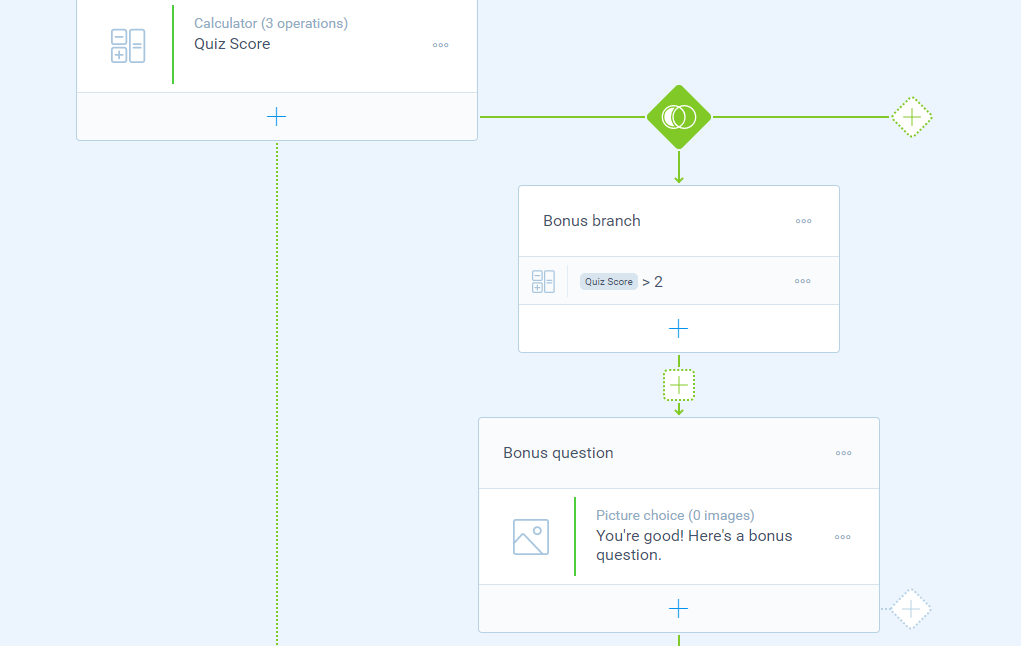How to Set Up a Calculator Form on Your WordPress Website - A Complete Guide With Examples Background story10 Min.

# How to Set Up a Calculator Form on Your WordPress Website - A Complete Guide With Examples

Calculations can help you build all sorts of form types. This post will show you how to set up a calculator form on your site using Tripetto!

In the past, forms could be simple elements of a site. However, now they are versatile and powerful, with lots of extra functionality. This can give you a better way to connect with users and provide a greater experience. A calculator is a fantastic way to help you apply discount codes, calculate scores, and more. While WordPress doesn’t have native form functionality or templates, a solution such as Tripetto does.

With Tripetto’s Calculator block, you can include multiple calculations in a single WordPress form. You can also get as advanced as you need to, as certain question blocks can also carry out complex and instant calculations for you. Even better, you can achieve all of this without the need for HTML, CSS, JavaScript, or any other code.

For this article, we’re going to show you how to use the Calculator block within Tripetto, and what sets it apart from the competition.

## Why You’d Want to Use Calculator Forms (And When To Do So)

While lots of businesses won’t need anything more than a simple WordPress form, in some cases, you’ll need to implement greater functionality. A more advanced form builder should include dedicated calculator functionality. With this you can create a number of powerful form types:

• Exam, quiz, and assessment forms, that calculates scores in real-time, and provides the results to other elements of your forms.
• Quote and order forms, which lets the calculator combine multiple form elements. For example, you can tally a running total, then apply a discount percentage based on user input.
• Health wizards – and not just for Body Mass Indicator (BMI) calculations, but for macronutrients, and other popular formulas.

For example, you can use a simple formula to calculate discount codes which will provide value and increase the effectiveness of your form:

You can take things even further though, almost to any level you need. While for example a BMI calculator isn’t something every site needs, the underlying principles are something you can adapt to any number of situations:A Tripetto form that calculates a BMI and gives an advice based on the calculation outcome.

However, there is much more you can do with dedicated calculator functionality for your forms:

• The most obvious is to use standard operators, such as add, subtract, multiple, and divide.
• You’re able to set fixed numbers, but more importantly use given answers from the respondent to other questions in the form, right inside your calculations.
• To easily calculate with selected options in the form, you should be able to add scores to each of the options in your form.
• You should also be able to compare values, whether that’s something calculated in real-time, or fixed.
• There should be the ability to set constants, such as a time or date, or apply mathematical constants wherever you need.
• In advanced use cases, the presence of more advanced mathematical functions will also let you develop complex and unique calculations.
• Calculating a desired outcome is one thing, but using the outcome so you can add the right conclusions to it, is just as important.

Overall, calculations are vital when you want to create dynamic content that boosts the User Experience (UX). To implement them, you’ll want to turn to a top-tier solution.

## Introducing Tripetto’s Calculator Block

By default, WordPress doesn’t include any functionality to display a form. In most circumstances, you would turn to a number of other plugins to help you add a form to your site.

However, Tripetto offers a bunch of stellar features to add forms to your WordPress site, one of which is the Calculator block. This starts with a core base of typical and straightforward operations:

From there, you can begin to play and perform the calculations you need for each use case. For example, you can use given answers and/or apply scores to the given answers from other questions in your form and do all kinds of calculations with them.

To take this a step further, you can also use advanced operations such as roots, powers, constants, functions, and more. This will be enough for most cases and already lets you create very powerful calculations within your forms.

However, you can also carry out subcalculations: This means calculations within calculations. Examples of this are checkout subtotals on order forms, tax calculations, and more. Further to this, you can also use multiple calculators in one form. A typical use case here is where you need to make a few different calculations in one form.

Because calculations in Tripetto are so flexibel, you can even use the outcome of one calculator as the input for another calculator. To turn back to our BMI calculation form, you could expand the scope to also cover body fat, macronutrient intake, and more.

Last great thing is that calculators in Tripetto can be used in any part of your form. This enables you to show the outcome to your respondents at any given position in your form, but you can even use the outcome to work with your logic flows. That way you can for example differentiate the route in your form that each respondent takes, based on the outcome of a calculator.

## How to Set Up and Use a Calculator Form With Tripetto

Over the rest of this article, we’re going to show you how to add and use a calculator form. If you can use WordPress, you can add this powerful Tripetto feature. We’re going to assume that you already have a Pro version of Tripetto, and that it’s installed and activated.

#### 📌 Also see: Instant calculations

Note that you don’t even need to work with the full Calculator block in some cases. We include some of the more common calculations within certain Question Blocks for instant calculations. For example, you can complete a running tally of scores within multiple choice blocks instantly. However, once you add a new Calculator block to your form you have full power inside that Calculator block.

The calculator is available as a question type in Tripetto's form builder. After you added a new block to your form, you can select the "Calculator" from the Question Type menu.

From here, you can give your Calculator block a description, so you can understand it at a glance later on.

### Step 2. Work With the Calculator’s Operations and Features

Given the scope of the included operations within Tripetto, it’s understandable that there will be lots to uncover. In simple terms, there are three elements to any calculation:

• Initial value. This is the starting point of your calculation. That can be a fixed number, but also a score or a given answer right away.
• Operation(s). Next, you supply the operation(s) you want to perform after the initial value, such as add, subtract, multiply or divide. Each operation is a step in your calculation.
• Operation properties. For each operation there are lots of options to perform the right calculation. You have all kinds of options that help you to use the right values with that, like a fixed value, a given answer, a score or a comparison.

With these elements you build up your calculation. For example, consider a three-question quiz, in which you want to calculate the score for each respondent, based on the given answers:

You can click each row in the calculator to open the operation's properties. For example to determine the scores of each option in a multiple choice question:

### Step 3. Run Through the Calculator Block’s Settings and Options

There are lots of options and settings for each Calculator block to help you format the outcome. You’ll find these at the left-hand side of each Calculator block in the form builder.

To demonstrate this, let's look at an order form, in which you want to calculate the total price of the entered amount of products. You want to show a dollar sign and the price must be calculated with 2 decimals. And you want a minimum purchase of \$9.99 and a maximum purchase of \$100.00. To achieve this, simply enable the needed options and configure them like you want:Configure the options of Tripetto's Calculator block to get the total price.

### Step 4. Using Logic With Your Calculator Block’s Outcomes

For a form that’s dynamic and adaptive, conditional logic is vital. Tripetto includes powerful logic options by default. What’s more, you can leverage this logic to work with your calculator outcomes. You can use this in a couple of ways:

• As a condition for a branch. This is akin to an ‘if statement’, which you can use to run a certain follow-up if the calculator returns a certain value.
• Within logic branches. If the conditions match, you’ll be able to perform calculations. For example, this could be as simple as detecting if a user populates two text fields. From here, you can look to iterate through and find multiple items and calculate subtotals (and more). You’ll then get a grand total at the end of the branch from the calculator.

There are lots of options to set branch conditions that extend the operations you carry out on your form calculators. For example, you can set conditions that check whether the calculation is equal or not equal to, higher or lower, between or in between, and much more.Use the outcome of a Calculator block to follow a logic flow (show the bonus question if the score is higher than 2).

We have a dedicated page within our Help Center on using logic within your calculations, and it’s essential reading to harness the power this provides.

## The Calculator Block Is Not All Tripetto Can Do

One of the standout features of the Tripetto WordPress plugin is the Calculator block. This can take a typical form and turn it into a supercharged, dynamic, and valuable experience on your website. However, it’s not the only string to Tripetto’s bow. There is lots more in the box: